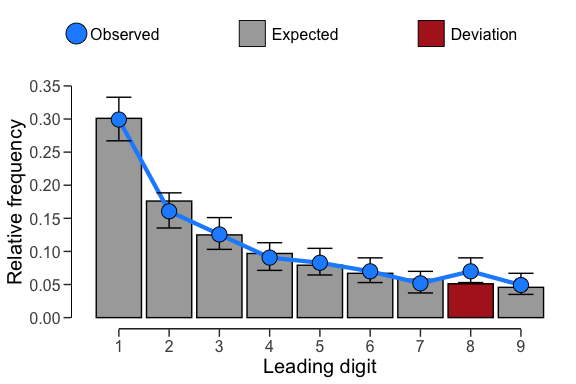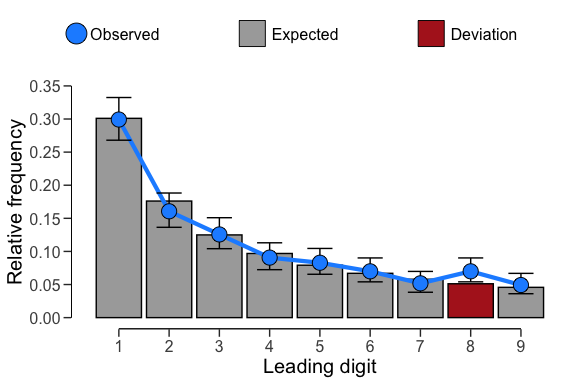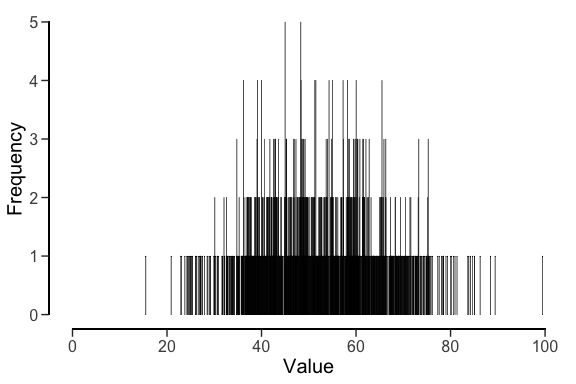# Digit analysis

## Introduction

Welcome to the ‘Digit analysis’ vignette of the jfa package. In this vignette you are able to find detailed examples of how you can incorporate the digit_test() and repeated_test() functions provided by the package.

## Function: digit_test()

The function digit_test() takes a vector of numeric values, extract the requested digits, and compares the frequencies of these digits to a reference distribution. The function either performs a frequentist hypothesis test of the null hypothesis that the digits are distributed according to the reference distribution and produces a p value or a Bayesian hypothesis test of the null hypothesis that the digits are distributed according to the reference distribution against the alternative hypothesis (using the prior parameters specified in prior) that the digits are not distributed according to the reference distribution and produces a Bayes factor (Kass & Raftery, 1995).

Example:

Benford’s law (Benford, 1938) is a principle that describes a pattern in many naturally-occurring numbers. According to Benford’s law, each possible leading digit d in a naturally occurring, or non-manipulated, set of numbers occurs with a probability p(d) = log10(1 + 1/d). The distribution of leading digits in a data set of financial transaction values (e.g., the sinoForest data) can be extracted and tested against the expected frequencies under Benford’s law using the code below.

# Frequentist hypothesis test
x <- digit_test(sinoForest$value, check = "first", reference = "benford") print(x) ## ## Digit Distribution Test ## ## data: sinoForest$value
## n = 772, MAD = 0.0065981, X-squared = 7.6517, df = 8, p-value = 0.4682
## alternative hypothesis: leading digit(s) are not distributed according to the benford distribution.
plot(x)# Bayesian hypothesis test using default prior
x <- digit_test(sinoForest$value, check = "first", reference = "benford", prior = TRUE) print(x) ## ## Digit Distribution Test ## ## data: sinoForest$value
## n = 772, MAD = 0.0065981, BF10 = 1.4493e-07
## alternative hypothesis: leading digit(s) are not distributed according to the benford distribution.
plot(x)## Function: repeated_test()

The function repeated_test() analyzes the frequency with which values get repeated within a set of numbers. Unlike Benford’s law, and its generalizations, this approach examines the entire number at once, not only the first or last digit. For the technical details of this procedure, see Simohnsohn (2019).

Example:

In this example we analyze a data set from a (retracted) paper that describes three experiments run in Chinese factories, where workers were nudged to use more hand-sanitizer. These data were shown to exhibited two classic markers of data tampering: impossibly similar means and the uneven distribution of last digits (Yu, Nelson, & Simohnson, 2018). We can use the repeated_test() function to test if these data also contain a greater amount of repeated values than expected if the data were not tampered with.

x <- repeated_test(sanitizer$value, check = "lasttwo", samples = 2000) print(x) ## ## Repeated Values Test ## ## data: sanitizer$value
## n = 1600, AF = 1.5225, p-value = 0.0035
## alternative hypothesis: average frequency in data is greater than for random data.
plot(x)